# Shafarevich conjecture

(diff) ← Older revision | Latest revision (diff) | Newer revision → (diff)

in inverse Galois theory

The absolute Galois group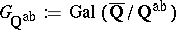of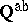(cf. also Galois group) is a free profinite group of countable rank. Here,is the maximal Abelian extension of, or, equivalently (by the Kronecker–Weber theorem), the maximal cyclotomic extension of.

I.R. Shafarevich posed this assertion as an important problem during a 1964 series of talks at Oberwolfach on the solution to the class field tower problem (cf. Tower of fields; Class field theory). The conjecture would imply an affirmative answer to the inverse Galois problem over, i.e. that every finite group is a Galois group over(cf. also Galois theory, inverse problem of). By the Iwasawa theorem [a7], p. 567, (see also [a1], Cor. 24.2), a profinite group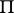of countable rank is free (as a profinite group) if and only if every finite embedding problem forhas a proper solution. Thus, the Shafarevich conjecture is equivalent to the assertion that ifis a quotient of a finite group, then every-Galois field extension ofis dominated by a-Galois field extension of.

A weakening of this assertion is known: that the profinite group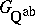is projective, i.e. every finite embedding problem forhas a weak solution (cf. also Projective group). Projectivity is equivalent to the condition of cohomological dimension[a12], Chap. 1; Props. 16, 45, and this holds forby [a12], Chap. 2; Prop. 9. On the other hand, the absolute Galois group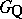is not projective, since the surjectioncorresponding to the extensiondoes not factor through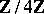. Thus, the analogue of the Shafarevich conjecture does not hold for.

## Evidence for the conjecture.

Many finite groups, including "most" simple groups, have been realized as Galois groups over[a9], Chap. II, Sec. 10. These realizations provide evidence for the inverse Galois problem overand hence for the Shafarevich conjecture. Typically, these realizations have been achieved by constructing Galois branched covers of the projective line over. Sinceis Hilbertian [a13], Cor. 1.28, such a realization implies that the covering group is a Galois group of a field extension of. Most of these branched covers have been constructed by means of rigidity; cf. [a9] and [a13] for a discussion of this approach. (Some of these covers are actually defined over the-line, and their covering groups are thus Galois groups over.)

The rigidity approach also suggests a possible way of proving the Shafarevich conjecture. B.H. Matzat introduced the notion of GAR-realizability of a group, this being realizability as the Galois group of a branched cover with certain additional properties (cf. [a9], Chap. 4, Sec. 3.1). Many simple groups have been GAR-realized over, and the Shafarevich conjecture would follow if it were shown that every finite simple group has a GAR-realization over. See [a9], Chap. 4; Sec. 3, 4.

The solvable case of the Shafarevich conjecture has been proven: K. Iwasawa [a7] showed that the maximal pro-solvable quotient ofis a free pro-solvable group of countable rank. In particular, every finite solvable group is a Galois group over, and every embedding problem forwith finite solvable kernel has a proper solution. Iwasawa's result also holds for the maximal Abelian extension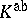of any global field, and for the maximal cyclotomic extension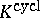of any global field[a7], Thm. 6, 7.

## Generalizations.

The Shafarevich conjecture can be posed withreplaced by any global field. In this generalized form, it asserts that the absolute Galois group ofis free of countable rank (as a profinite group). This conjecture remains open (as of 2001) in the number field case, but has been proven by D. Harbater [a6], Cor. 4.2, and F. Pop [a10] in the case thatis the function field of a curve over a finite field. (See also [a5], Cor. 4.7, and [a9], Sec. V.2.4.) Sinceifis a finite field of characteristic, this assertion is equivalent to stating that the absolute Galois group ofis free of countable rank ifis the function field of a curve over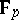. This result is shown by using patching methods involving formal schemes or rigid analytic spaces, in order to show that all finite embedding problems for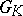have a proper solution — i.e. that every connected-Galois branched cover of the curve is dominated by a connected-Galois branched cover, ifis a quotient of the finite group. By Iwasawa's theorem [a7], p. 567, the result follows. The proof also shows that ifis a curve over an arbitrary algebraically closed field of cardinality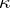, and ifis the function field of, then every finite embedding problem forhas exactlyproper solutions. By the Mel'nikov–Chatzidakis theorem [a8], Lemma 2.1, it follows thatis free profinite of rank, generalizing the geometric case of the Shafarevich conjecture (see [a6], Thm. 4.4, [a10], Cor. to Thm. A).

As another proposed generalization of the Shafarevich conjecture (which would subsume the above case of global fields), M. Fried and H. Völklein conjectured [a2], p. 470, that ifis a countable Hilbertian field whose absolute Galois groupis projective, thenis free of countable rank. They proved a special case of this [a2], Thm. A, viz. thatis free of countable rank ifis a countable Hilbertian pseudo-algebraically closed field (a PAC field) of characteristic. For example, this applies to the field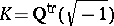, where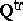is the field of totally real algebraic numbers, by results of R. Weissauer and Pop; see [a13], p. 151, [a9], p. 286. Later, Pop [a11], Thm. 1, removed the characteristichypothesis from the above result. This solves a problem in [a1], Problem 24.41. (See also [a4].) Sinceis not PAC (as proven by G. Frey [a1], Cor.10.15), this result does not prove the Shafarevich conjecture itself. But it does imply thathas a free normal subgroup of countable rank for which the quotient is of the form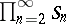[a2] (instead of the formas in the Shafarevich conjecture). The above Fried–Völklein conjecture holds ifis Galois over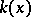, foran algebraically closed field ([a8], Prop. 4.4, using the geometric case of the Shafarevich conjecture [a6], [a10]). More generally, it holds ifis large in the sense of Pop [a11], Thm. 2.1; cf. also [a9], Sec. V.4. A solvable case of the conjecture holds, extending Iwasawa's result: ForHilbertian withprojective, every embedding problem forwith finite solvable kernel has a proper solution [a13], Cor. 8.25.

How to Cite This Entry:
Shafarevich conjecture. Encyclopedia of Mathematics. URL: http://encyclopediaofmath.org/index.php?title=Shafarevich_conjecture&oldid=14221
This article was adapted from an original article by David Harbater (originator), which appeared in Encyclopedia of Mathematics - ISBN 1402006098. See original article